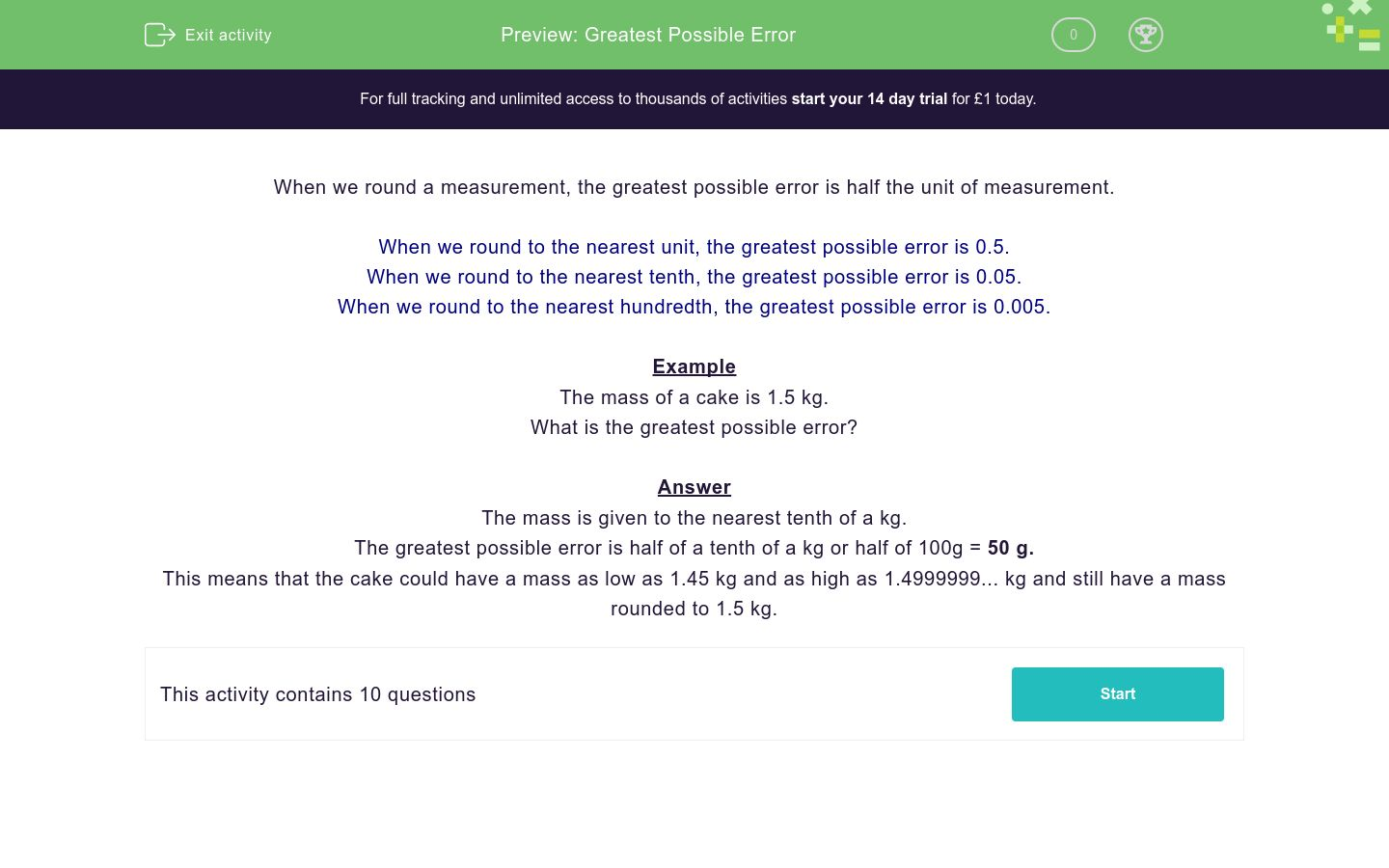# Greatest Possible Error

In this worksheet, students must state the largest possible error when a measurement or number is given.Key stage:  KS 4

Curriculum topic:  Number

Curriculum subtopic:  Apply/Interpret Limits of Accuracy When Rounding/Truncating

Difficulty level:### QUESTION 1 of 10

When we round a measurement, the greatest possible error is half the unit of measurement.

When we round to the nearest unit, the greatest possible error is 0.5.

When we round to the nearest tenth, the greatest possible error is 0.05.

When we round to the nearest hundredth, the greatest possible error is 0.005.

Example

The mass of a cake is 1.5 kg.

What is the greatest possible error?

The mass is given to the nearest tenth of a kg.

The greatest possible error is half of a tenth of a kg or half of 100g = 50 g.

This means that the cake could have a mass as low as 1.45 kg and as high as 1.4999999... kg and still have a mass rounded to 1.5 kg.

The mass of a cake is 1.3 kg.

What is the greatest possible error?

1 kg

100 g

50 g

10 g

5 g

The mass of a loaf of bread is 2 kg.

What is the greatest possible error?

1 kg

100 g

50 g

10 g

500 g

The mass of a loaf of bread is 2.25 kg.

What is the greatest possible error?

1 kg

100 g

50 g

10 g

5 g

A table is 3 metres long.

What is the greatest possible error?

1 m

50 cm

10 cm

5 cm

5 mm

A table is 3.4 metres long.

What is the greatest possible error?

1 m

50 cm

10 cm

5 cm

5 mm

A table is 3.47 metres long.

What is the greatest possible error?

1 m

50 cm

10 cm

5 cm

5 mm

A table is 3.0 metres long.

What is the greatest possible error?

1 m

50 cm

10 cm

5 cm

5 mm

There are 2 litres of water in a bottle.

What is the greatest possible error?

1 l

500 ml

100 ml

50 ml

5 ml

There are 2.0 litres of water in a bottle.

What is the greatest possible error?

1 l

500 ml

100 ml

50 ml

5 ml

A table is 152.4 cm long.

What is the greatest possible error?

0.5 mm

500 mm

0.5 cm

50 mm

5 mm

• Question 1

The mass of a cake is 1.3 kg.

What is the greatest possible error?

50 g
EDDIE SAYS
Greatest possible error is half of 0.1 kg.
• Question 2

The mass of a loaf of bread is 2 kg.

What is the greatest possible error?

500 g
EDDIE SAYS
Greatest possible error is half of 1 kg.
• Question 3

The mass of a loaf of bread is 2.25 kg.

What is the greatest possible error?

5 g
EDDIE SAYS
Greatest possible error is half of 0.01 kg, which is half of 10 g.
• Question 4

A table is 3 metres long.

What is the greatest possible error?

50 cm
EDDIE SAYS
Greatest possible error is half of 1 m.
• Question 5

A table is 3.4 metres long.

What is the greatest possible error?

5 cm
EDDIE SAYS
Greatest possible error is half of 0.1 m, which is half of 10 cm.
• Question 6

A table is 3.47 metres long.

What is the greatest possible error?

5 mm
EDDIE SAYS
Greatest possible error is half of 0.01 m, which is half of 1 cm.
• Question 7

A table is 3.0 metres long.

What is the greatest possible error?

5 cm
EDDIE SAYS
The first decimal place is given as 0, so this is measured to the nearest tenth of a metre.
Greatest possible error is half of 0.1 m.
• Question 8

There are 2 litres of water in a bottle.

What is the greatest possible error?

500 ml
EDDIE SAYS
Greatest possible error is half of 1 l.
• Question 9

There are 2.0 litres of water in a bottle.

What is the greatest possible error?

50 ml
EDDIE SAYS
Greatest possible error is half of 100 ml.
• Question 10

A table is 152.4 cm long.

What is the greatest possible error?

0.5 mm
EDDIE SAYS
Greatest possible error is half of 0.1 cm, which is half of 1 mm.
---- OR ----

Sign up for a £1 trial so you can track and measure your child's progress on this activity.

### What is EdPlace?

We're your National Curriculum aligned online education content provider helping each child succeed in English, maths and science from year 1 to GCSE. With an EdPlace account you’ll be able to track and measure progress, helping each child achieve their best. We build confidence and attainment by personalising each child’s learning at a level that suits them.